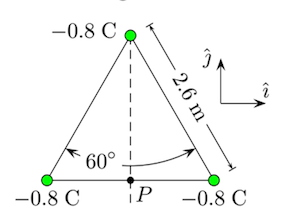# Problem: Three point charges are placed at the vertices of an equilateral triangle. Find the magnitude of the electric field E at P. The value of the Coulomb constant is 8.9875 × 109 N · m2/C2. Answer in units of N/C.

🤓 Based on our data, we think this question is relevant for Professor Markert's class at TEXAS.

###### Problem Details

Three point charges are placed at the vertices of an equilateral triangle. Find the magnitude of the electric field E at P. The value of the Coulomb constant is 8.9875 × 109 N · m2/C2. Answer in units of N/C.